## Welcome to the Second Life Forums Archive

These forums are CLOSED. Please visit the new forums HERE

# discussion Binary Math

MC Seattle
Registered User
Join date: 3 Apr 2006
Posts: 63
04-14-2006 03:36
CODE
`// LSL Binary Arithmetic// (Anti-)Proof of concept//// The largest numbers LSL supports are 32-bit signed // integers, which limits llModPow to 16-bit exponents, // therefore limiting key length in RSA encryption and // Diffie-Hellman exchanges to 16-bit keys. To get // around this limitation I started writing a binary // arithmetic library which would allow arbitrary size // numbers in ModPow functions, bringing LSL up to // par with the strength of PHP libraries like bcmath and // gmp. Unfortunately, the speed of LSL makes this a // laughable experience. Multiplying the two 32-bit // test numbers takes over 30 seconds in my tests. I // guess 64-bit exponents are out of the question...//     (What was I thinking?)// Anyways instead of throwing the code away I'm // archiving it here in case anyone else gets the wild // idea to try something similar. Addition and subtraction // can be done very quickly if someone has a use for those, // and this could probably be optimized by using strings // instead of lists. Only addition and multiplication are // implemented in this version, but from my testing they // are both very robust. Unless you start passing non-// binary data in of course. // Some random test values, 3816682369 and 2305728161list number_one = [1,1,1,0,0,0,1,1,0,1,1,1,1,1,0,1,1,1,1,1,0,0,1,1,1,0,0,0,0,0,0,1];list number_two = [1,0,0,0,1,0,0,1,0,1,1,0,1,1,1,0,1,0,0,1,1,1,1,0,1,0,1,0,0,0,0,1];print_binary(list number){    integer i;    string output;        for (i = 0; i < llGetListLength(number); i++) {        output += llList2String(number, i);    }        llSay(0, output);}list reverse(list number){    list new;    integer i;        for (i = llGetListLength(number) - 1; i >= 0; i--) {        new += llList2List(number, i, i);    }        return new;}list add(list first, list second){    list answer = [];    list temp = [];    integer i;    integer f;    integer s;    integer carry = FALSE;    integer diff = llGetListLength(first) - llGetListLength(second);    // Pad the one of the numbers with leading zeroes if necessary       if (diff < 0) {        for (i = 0; i < llAbs(diff); i++) {            temp += ;        }                first = temp + first;    } else if (diff > 0) {        for (i = 0; i < diff; i++) {            temp += ;        }                second = temp + second;    }        // Addition loop    for (i = llGetListLength(first) - 1; i >= 0; i--) {        f = llList2Integer(first, i);        s = llList2Integer(second, i);        if (f && s && carry) {            carry = TRUE;            answer += ;        } else if (f && s || ((f || s) && carry)) {            carry = TRUE;            answer += ;        } else {            if (f || s || carry) {                answer += ;            } else {                answer += ;            }                        carry = FALSE;        }    }        if (carry) {        answer += ;    }        return reverse(answer);}list multiply(list multiplicand, list multiplier){    integer i;    integer j;    list partial_sum = ;    list product;    integer length = llGetListLength(multiplier);        for (i = length - 1; i >= 0; i--) {        product = [];                if (llList2Integer(multiplier, i)) {            // Build the right-side zero padding for the product            for (j = 0; j < (length - i) - 1; j++) {                product += ;            }                        product = multiplicand + product;            partial_sum = add(partial_sum, product);        }    }        return partial_sum;}default{    state_entry()    {    }    touch_start(integer total_number)    {        list answer;                llSay(0, "Free memory: " + (string)llGetFreeMemory());                llSay(0, "Binary addition test");        print_binary(number_one);        print_binary(number_two);        llSay(0, "--------------------------------");        answer = add(number_one, number_two);        print_binary(answer);                llSay(0, "Binary multiplication test");        print_binary(number_one);        print_binary(number_two);        llSay(0, "--------------------------------");        answer = multiply(number_one, number_two);        print_binary(answer);                llSay(0, "Free memory: " + (string)llGetFreeMemory());    }}`
Nada Epoch
The Librarian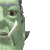Join date: 4 Nov 2002
Posts: 1,423
Original Thread
04-14-2006 09:02
/15/2c/100205/1.html
_____________________
i've got nothing.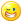OneBigRiver Stork
Diversity matters
Join date: 29 Mar 2006
Posts: 44
04-14-2006 16:23
From: MC Seattle
CODE
`list number_one = [1,1,1,0,0,0,1,1,0,1,1,1,1,1,0,1,1,1,1,1,0,0,1,1,1,0,0,0,0,0,0,1];list number_two = [1,0,0,0,1,0,0,1,0,1,1,0,1,1,1,0,1,0,0,1,1,1,1,0,1,0,1,0,0,0,0,1];`

The problem with this code is that each "bit" is being represented by a 32-bit integer... a big waste! You should definitely use at least 30 bits for each entry (32nd bit is the sign bit, might be good to stay away. If you also keep the 31st bit at 0, this will facilitate carries).

I have been thinking about this too, so maybe I will code something up quick and see if I can make it work.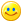OneBigRiver Stork
Diversity matters
Join date: 29 Mar 2006
Posts: 44
04-14-2006 18:03
From: OneBigRiver Stork
The problem with this code is that each "bit" is being represented by a 32-bit integer... a big waste! You should definitely use at least 30 bits for each entry (32nd bit is the sign bit, might be good to stay away. If you also keep the 31st bit at 0, this will facilitate carries).

I have been thinking about this too, so maybe I will code something up quick and see if I can make it work.Well, 60 bits works pretty well. For giggles I tried to get up to 1000 bits (the range of RSA keys I feel happy with). I tried a normal linear add, and it was terrible. Then I tried using a recursive add (split the number in half, add the halves independantly, then concatenate at the end). My hope is that this would eliminate a lot of list traversal badness, but it didn't seem to help.

Does anyone know how lists are really implemented under the hood? Anyway, it was kindof an academic exercise anyway. It is pretty hard to deal with 1024-bit keys even with native code, so we probably won't get good encryption unless the Lindens provide it.Strife Onizuka
Moonchild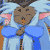Join date: 3 Mar 2004
Posts: 5,887
04-14-2006 19:39
I'd be much more interested if someone would implement a float library.

BTW you can write your print_binary function as
print_binary(list a){llSay(0, (string)a);}

remember LSL does not optimize your code in any way at all (it is a scripting language).
_____________________
Truth is a river that is always splitting up into arms that reunite. Islanded between the arms, the inhabitants argue for a lifetime as to which is the main river.
- Cyril Connolly

Without the political will to find common ground, the continual friction of tactic and counter tactic, only creates suspicion and hatred and vengeance, and perpetuates the cycle of violence.
- James Nachtwey
Merlin Alphabeta
Registered User
Join date: 12 Feb 2006
Posts: 83
04-19-2006 07:43
From: OneBigRiver Stork
Does anyone know how lists are really implemented under the hood? Anyway, it was kindof an academic exercise anyway. It is pretty hard to deal with 1024-bit keys even with native code, so we probably won't get good encryption unless the Lindens provide it.Keep in mind... CLR is coming... that should make all of this talk moot.

So, BTW, is llHttpRequest...

In the meantime if you want to do big number math, your best bet is to use 30-bit integers in a list, as someone else mentioned.

There, you'll have two problems:

1. Carries in radix-2 are non-intuitive to radix-10 users like us. Check your formulas carefully

2. There's really no good way to parse back and forth from strings easily. I've done it before (and have some VB code I could dig up if you really want it - IM me in world if interested) The way to solve this problem is with TWO math libraries - one that works on binary lists, and one that works directly on strings. So you could have an add method that looks like (pseudocode)

integer carry = 0
for(i=end of string; i>=0; i--) {
integer amount = (substring(str1, i, i) - "0"+ (substring(str2, i, i) - "0"+ carry
carry = (integer)(amount / 10)
amount -= (carry * 10)
resultstring = (string)amount + resultstring
}

with appropriate math functions then you will have a series of functions that manipulates ascii-encoded strings - from there it's trivial to do binary translation. Basically you just keep comparing to succesively smaller powers of two - for any one that is greater than you current number, you subtract it from the number and turn that bit on in your result.
grumble Loudon
A Little bit a lion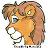Join date: 30 Nov 2005
Posts: 612
05-01-2006 21:53
I would store the numbers 16 bits at a time in reverse. This would allow the mutiply to use the mutiply operator. Internaly Lists are a series of pointers to objects so it's important not to copy or pass them to functiuons since they and there data is copyed each time. I heard each object is like 64 bytes regaurdless of what type it is.

Use global lists.

//32 bit Unsigned multiply example.
//  is the LSB
ListOut = List1 * List2 ' low * low
ListOut += List1 * List2 ' low * high
ListOut += List1 * List2 ' High * low
ListOut += List1 * List2 ' High * high

// add carrys starting at bottom
ListOut += ListOut / 0x1000
ListOut += ListOut / 0x1000

//loop

//Signed mutiplys requre removing the sign bits and then reapplying them afterwards.

I have written 64 bit divide routines on 8 bit processors, so I can tell you that it is posible.

If you need a Square root, use the binary test method where you do one mutiply per output bit.

Also Don't modify the list more than you have to!
Since there is no llListChangeEntry the system copys the entire list each time the list entrys are updated.

This means that you need to cache the 3 list entrys and then save [i+0] as you work your way up the list.

edit: "/16" should be ">> 16" or "/ 0x1000"
Also since SL uses signed integers, you must do the carry after each mutiply and not after every two mutiplys.
Merlin Alphabeta
Registered User
Join date: 12 Feb 2006
Posts: 83
05-11-2006 09:51
divides are tough - I usually use iterative subtraction and just avoid divides like the plague in my code.

The multiply code could be better written as (16-bit integers)

I don't know if this is 2's-compliment sign compatible or not. I'm guessing it is, since that's the reason we use 2's compliment instead of 1's compliment for signs - it's supposed to make all per-word and per-bit mathematical operations compatible. I'm basically simulating a 16-bit word and 16-bit carry here, just like you suggested, so that should be compatible.

No way to know without trying it...

list multiply(list a, list b) {
list out;
integer i;
integer j;
for(i=0; i<llGetListLength(a); i++) {
integer carry = 0;
for(j=0; j<llGetListLength(b); j++) {
integer result = llList2Integer(a, i) * llList2Integer(b, j) + llList2Integer(out, i+j);
carry = (integer)(result / 0x10000);
result -= carry * 0x10000;
out = llListReplaceList(out, [ result ], i+j, i+j);
}
if(carry != 0) {
// this is a simplification of (length(a) - 1) + (length(b) - 1) + 1
integer carrydigit = llGetListLength(a) + llGetListLength(b) - 1;
while(carry != 0) {
integer result = llList2Integer(out, carrydigit) + carry;
carry = (integer)(result / 0x10000);
result -= carry * 0x10000;
out = llListReplaceList(out, [ result], carrydigit, carrydigit);
carrydigit++;
}
}
}
}
010000100111001001100001011011 Omlet
Game Art and Design Stud.
Join date: 20 Feb 2006
Posts: 10
pfff
07-12-2006 12:04
Binary sucks....

p.s.

good job tho XD
Ordinal Malaprop
really very ordinary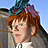Join date: 9 Sep 2005
Posts: 4,607
07-12-2006 12:07
01000100011011110110111000100111011101000010000001100010011001010010000001100001001000000110010001101001011000110110101100101110
Bitzer Balderdash
Dazed and Confused
Join date: 21 Dec 2005
Posts: 246
07-13-2006 01:42
From: Ordinal Malaprop
01000100011011110110111000100111011101000010000001100010011001010010000001100001001000000110010001101001011000110110101100101110

LMAO

(the scary part is that I didn't need to use an ascii table to convert this - just a text editor to split it into bytes O.o)
Draco18s Majestic
Registered User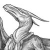Join date: 19 Sep 2005
Posts: 2,744
07-13-2006 07:01
From: Bitzer Balderdash
LMAO

(the scary part is that I didn't need to use an ascii table to convert this - just a text editor to split it into bytes O.o)

I could have done that and then dug up my Assembler book and done it too, but it's in a difference city than I am, so....I just used and online too.
010000100111001001100001011011 Omlet
Game Art and Design Stud.
Join date: 20 Feb 2006
Posts: 10
02-23-2007 14:47
From: Ordinal Malaprop
01000100011011110110111000100111011101000010000001100010011001010010000001100001001000000110010001101001011000110110101100101110

aww...sorry...